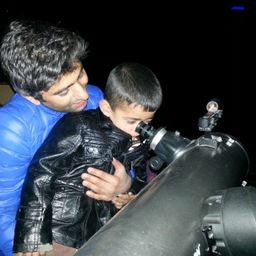## 19m

Gravitation

### Discussion

You must be signed in to discuss.
##### Top Physics 101 Mechanics EducatorsLB##### Marshall S.

University of Washington##### Aspen F.

University of Sheffield

Lectures

Join Bootcamp

### Video Transcript

using L physical to proper lend times squared Rudolph one minus well, we squared, divided by cease were well in this case, proper land is equal to 100 meters, 100 meters and therefore angle is equal to we're also even be. We is equal to zero point buying five times the speed of light. So Al is equal to 100. Multiply by squared Rudolph one minus hoese Tzeitel point 95 times c all square divided by C square and L is equal to 100. Um, multiply by one minus 0.95 whole square, zero point mine by all square and this gives us 90 meters.#### Topics

Gravitation

##### Top Physics 101 Mechanics EducatorsLB##### Marshall S.

University of Washington##### Aspen F.

University of Sheffield

Lectures

Join Bootcamp